• Save

# Problems on Ages with Solutions for SSC and Bank Exams

Last year 3.0K ViewsUsually, students have finds solutions of problems on ages because they face problems on ages questions in their competitive exams. But students are puzzled, where they should search problems on ages with solutions for ssc or bank exams.

So, here in this post, you can easily understand problems on ages with solutions and understand how to face and solve problems on ages. You can practice problems on ages questions and answers for competitive exams after learning to solve problems.

As well as you can know problems on ages tricks to solve these types of questions.

## Problems on Ages with Solutions for Competitive Exams

Q.1. Rajeev’s age after 15 years will be 5 time his age 5 years back. What is the present age of Rajeev?

Sol. Let Rajeev’s present age be x years. Then,

Rajeev’s age after 15 year = (x+15) years.

Rajeev’s age 5 years back = (x-5) years.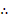x+15 = 5(x-5) ↔ x+15 = 5x – 25 ↔ 4x = 40 ↔ x = 10

Hence, Rajeev’s present age = 10 years.

Q.2. The product of the ages of Ankit and Nikita is 240. If twice the age of Nikita is more than Ankit’s age by 4 years, what is Nikita’s age?

Sol. Let Ankit’s age be x years. Then, Nikita’s age =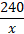years.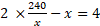↔ 480 – x2 = 4x ↔ x2 + 4x – 480 = 0

↔ (x + 24) (x – 20) = 0 ↔ x = 20

Hence, Nikita’s age =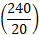years = 12 years.

Q.3. Rohit was 4 times as old as his son 8 years ago. After 8 years, Rohit will be twice as old as his son. What are their present ages?

Sol: Let son’s age 8 years ago be x years. Then, Rohit age 8 years ago = 4x years.

Son’s age after 8 years = (x+8) +8=(x+16) years.

Rohit age after 8 years = (4x+8) +8 = (4x+16) years.2(x+16) = 4x +16 ↔ 2x = 16 ↔ x = 8

Hence, son’s present age = (x + 8) = 16 years.

Rohit present age = (4x +8) = 40 years.

Q.4. Abhay’s age after six years will be three-seventh of his father’s age. Ten years ago, the ratio of their ages was 1: 5. What is Abhay’s father’s age at present?

Sol: Let the ages of ages of Abhay and his father 10 years ago be x and 5x years respectively. Then, Abhay’s age after 6 years = (x + 10) + 6 = (x + 16) years.

Father’s age after 6 years = (5x +10) +6= (5x + 16) years.(x+16) =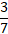(5x+16) ↔ 7(x+16) =3(5x+16) ↔ 7x + 112 = 15x + 48 ↔ 8x = 64 ↔ x = 8.

Hence, Abhay’s father’s present age = (5x +10) = 50 years.

Q.5. The ratio of the father’s age to his son’s age is 7: 3. The product of their ages is 756. The ratio of their ages after 6 years will be:

Solution: Let the present ages of the father and son be 7x and 3x years respectively.

Then, 7x × 3x = 756 ↔ 21x2 = 756 ↔ x2 = 36 ↔ x = 6Required ratio = (7x +6): (3x + 6) = 48: 24 = 2: 1.

Problems on ages

Q.6. The age of two persons differ by 16 years. If 6 years ago, the elder one be 3 time as old as the younger one, what is Nikita’s age?

Sol. Let the age of the younger person be x years.

Then, age of the elder person = (x+16) years.3 (x-6) = (x +16-6) ↔ 3x – 18 = x +10 ↔ 2x = 28 ↔ x = 14.

Hence, their present ages are 14 years and 30 years.

Q.7. The present age of a father is 3 years more than three times the age of his son. Three years hence, father’s age will be 10 years more than twice the age of the son. Find the present age of the father.

Sol: Let the son’s present age be x years. Then father’s present age = (3x +3) years.(3x+3+3) = 2 (x+3) +10 ↔ 3x +6 = 2x +16 ↔ x= 10.

Hence father’s present age = (3x +3) = (3 × 10 + 3) years = 33 years.

Q.8. One year ago, the ratio of Gaurav’s and Sachin’s age was 6: 7 respectively. Four years hence would become 7: 8. How old is Sachin?

Sol. Let Gaurav’s and Sachin’s ages one year ago be 6x and 7x years respectively. Then Gaurav’s age 4 year hence = (6x +1) + 4 = (6x + 5) years.

Sachin’s age 4 year hence = (7x+1) +4 = (7x +5) years.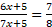↔ 8(6x+5) = 7(7x+5) ↔48x + 40 = 49x + 35 ↔ x = 5.

Hence, Sachin’s present age = (7x+1) = 36 years.

Q.9. Sachin is younger than Rahul by 4 years. If their ages are in the respectively ratio of 7 : 9, how old is Sachin ?

Sol: Let Rahul’s age be x years. Then, Sachin’s age = (x-7) years.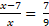↔ 9x – 63 = 7x ↔ 2x = 63 ↔ x = 31.5

Hence, Sachin age (x -7) = 24.5 years.

Q.10. The ratio of the ages of a man and his wife is 4 : 3. After 4 years, this ratio will be 9 : 7. If at the time of marriage, the ratio was 5 : 3, then how many years age were they married ?

Solution: Let the present ages of the man and his wife be 4x and 3x years respectively.

Then,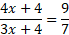↔ 7(4x + 4 ) = 9(3x+4) ↔ x = 8

So, their present ages are 32 years and 24 years respectively. Suppose they were married z years age.

Then,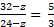↔ 3(32-z) = 5(24-z) ↔ 2z = 24 ↔ z = 12.

I hope these problems on ages with solutions will help you in your competitive exams to solving your questions correctly.  For more practice visit on the problems on ages questions with answers.

You can ask me anything in the comment section related problems on ages with solutions.

Best of luck for your exams